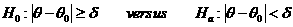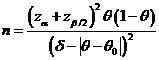# Sample Size Calculator: One Sample Proportion

Hypothesis: One-Sided EquivalenceData Input: (Help) (Example)

 Input Results ú\ ú] úc N úc0 ú_

Note:

 Variables Descriptions ú\ One-sided significance level 1-ú] Power of the test úc Expected success proportion of sample úc0 Known success proportion ú_ Equivalence limit N Sample size

Help Aids Top

Application: To establish equivalence, you can consider the following hypotheses:Procedure:

1. Enter

a)      value of ú\, the probability of type I error

b)      value of ú], the probability of type II error

c)      value of úc, a true mean response rate of a test drug

d)     value of úc0, a reference response rate

e)      value of ú_, the equivalence limit.

1. Click the button íºCalculateí¿ to obtain result sample size N.

Formula:(*)

Notations:

ú\:               The probability of type I error (significance level) is the probability of rejecting the true null hypothesis.

ú]:               The probability of type II error (1 íV power of the test) is the probability of failing to reject the false null hypothesis.

úc-úc0:          The difference between the true mean response rates of a test drug (úc) and a reference value (úc0).

ú_:               Clinically meaningful difference. The largest change from the reference value (baseline) that is considered to be trivial.

Examples

Example 1: We consider that one brand name drug for osteoporosis on the market has a responder rate of 60%. It is believed that a ú_=20% difference in responder rate is of no clinical significance. Hence, the investigator wants to show the study drug is equivalent to the market drug in term of responder rate. If the true responder rate is úc0=65%, by (*), at ú\=0.05, assuming that the true response rate is úc=60%, then the required sample size for having an 80% power (i.e., ú]=0.2) is N=92.

Reference:

1. Casagrande, Pike and Smith (1978), Biometrics 34: 483-486.
2. Chow, Shao and Wang, Sample Size Calculations In Clinical Research, Taylor & Francis, NY. (2003) Pages 85-86.
3. Flesis J.L., Statistical Methods for Rates and Proportions (2nd edition). Wiley: New York, 1981.

Top